### TEST Statement

<label:> TEST equation1 <, equation2, …> </ option> ;

The TEST statement tests linear hypotheses about the regression coefficients. The Wald test is used to jointly test the null hypotheses (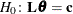) specified in a single TEST statement. When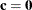you should specify a CONTRAST statement instead.

Each equation specifies a linear hypothesis (a row of the
matrix and the corresponding element of thevector); multiple equations are separated by commas. The label, which must be a valid SAS name, is used to identify the resulting output and should always be included. You can submit multiple TEST statements.

The form of an equation is as follows:

term <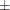term …> < =term <term …>>

where term is a parameter of the model, or a constant, or a constant times a parameter. For a binary response model, the intercept parameter is named INTERCEPT; for an ordinal response model, the intercept parameters are named INTERCEPT, INTERCEPT2, INTERCEPT3, and so on. When no equal sign appears, the expression is set to 0. The following illustrates possible uses of the TEST statement:

proc surveylogistic;
model y= a1 a2 a3 a4;
test1: test intercept + .5 * a2 = 0;
test2: test intercept + .5 * a2;
test3: test a1=a2=a3;
test4: test a1=a2, a2=a3;
run;


Note that the first and second TEST statements are equivalent, as are the third and fourth TEST statements.

You can specify the following option in the TEST statement after a slash (/):

PRINT

displays intermediate calculations in the testing of the null hypothesis. This includes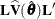bordered by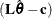and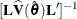bordered by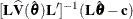, where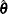is the pseudo-estimator ofand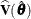is the estimated covariance matrix of.